# Math in Focus Grade 3 Chapter 15 Practice 1 Answer Key Measuring Length

This handy Math in Focus Grade 3 Workbook Answer Key Chapter 15 Practice 1 Measuring Length provides detailed solutions for the textbook questions.

## Math in Focus Grade 3 Chapter 15 Practice 1 Answer Key Measuring Length

Measure each to the nearest inch.

Example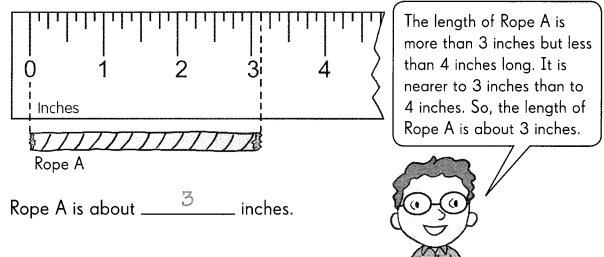Question 1.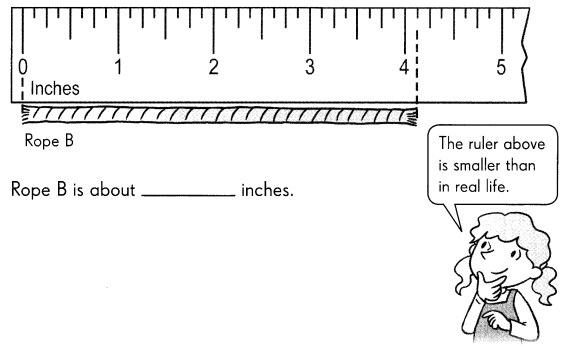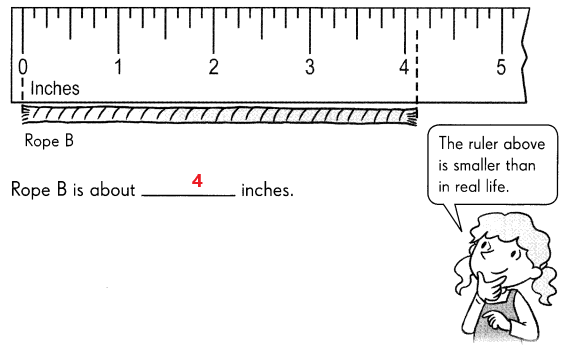Explanation:
In the above image we can observe Ruler and Rope B. The length of the Rope B is calculated by using Ruler.
The length of rope B is more than 4 inches but less than 5 inches long. It is nearer to 4 inches than to 5 inches. So, the length of Rope B is about 4 inches.

Question 2.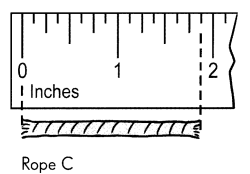Rope C is about ___________ inches.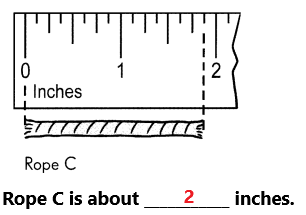Explanation:
In the above image we can observe Ruler and Rope C. The length of the Rope C is calculated by using Ruler.
The length of rope C is more than 1 inch long. It is nearer to 2 inches than 1 inch. So, the length of Rope C is about 2 inches.

Question 3.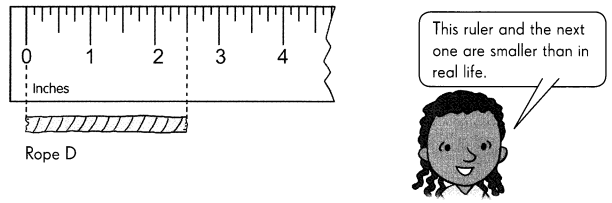Rope D is about ___________ inches.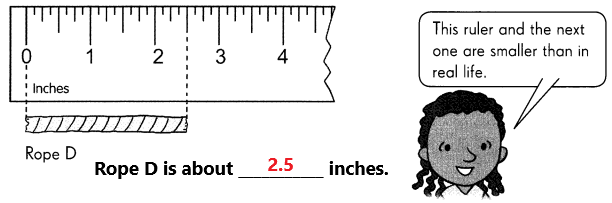Explanation:
In the above image we can observe Ruler and Rope D. The length of the Rope D is calculated by using Ruler.
The length of rope D is in between 2 and 3 inches. So, the length of Rope D is about 2.5 inches.

Measure each ribbon to the nearest half-inch.

Example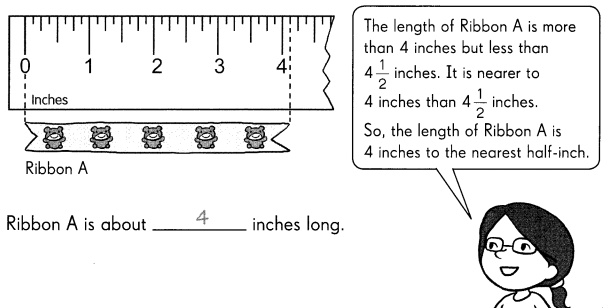Question 4.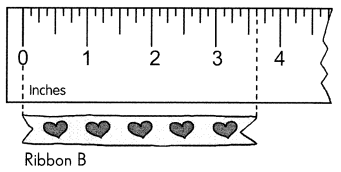Ribbon B is about __________ inches long.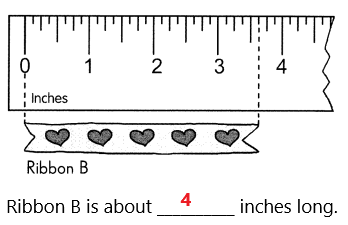Explanation:
In the above image we can observe Ruler and Ribbon B. The length of the Ribbon B is calculated by using Ruler.
The length of ribbon B is more than 3 1/2 inches. It is nearer to 4 inches than 3 inches. So, the length of Ribbon B is about 4 inches long.

Question 5.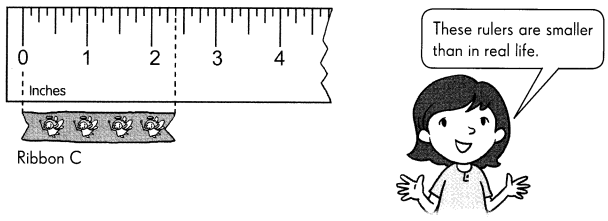Ribbon C is about __________ inches long.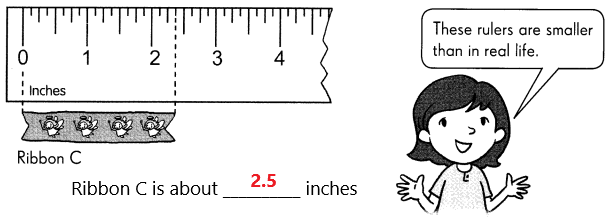Explanation:
In the above image we can observe Ruler and Ribbon C. The length of the Ribbon C is calculated by using Ruler.
The length of Ribbon C is more than 2 inches but less than 2 1/2 inches. It is nearer to 2 1/2 inches than 2 inches. So, the length of Ribbon C is about 2 1/2 inches long.

Question 6.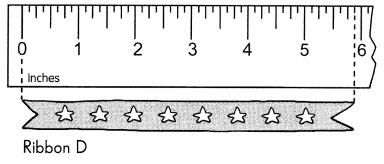Ribbon D is about __________ inches long.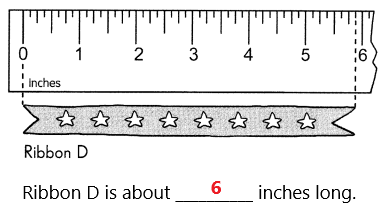Explanation:
In the above image we can observe Ruler and Ribbon D. The length of the Ribbon D is calculated by using Ruler.
The length of Ribbon D is more than 5 1/2 inches but less than 6 inches. It is nearer to 6 inches than 5 1/2 inches. So, the length of Ribbon D is about 6 inches long.

Question 7.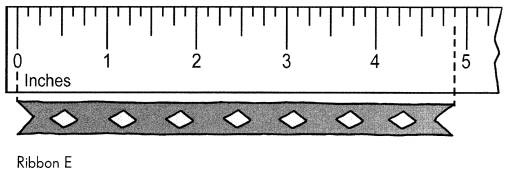Ribbon E is about __________ inches long.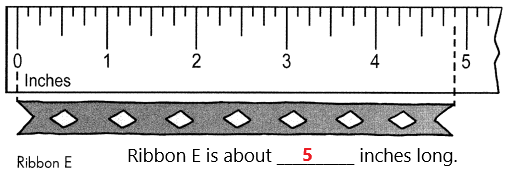Explanation:
In the above image we can observe Ruler and Ribbon E. The length of the Ribbon E is calculated by using Ruler.
The length of Ribbon E is more than 4 1/2 inches but less than 5 inches. It is nearer to 5 inches than 4 1/2 inches. So, the length of Ribbon E is about 5 inches long.

Question 8.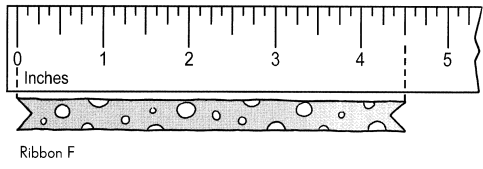Ribbon F is about __________ inches long.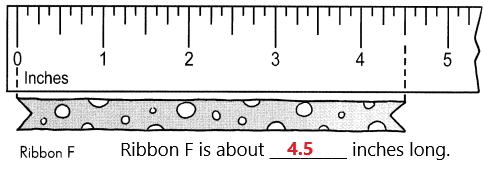Explanation:
In the above image we can observe Ruler and Ribbon F. The length of the Ribbon F is calculated by using Ruler
The length of Ribbon F is more than 4 inches but less than 5 inches. It is at 4 1/2 inches. So, the length of Ribbon F is about 4.5 inches long.

Estimate the length of each object to the nearest half-inch.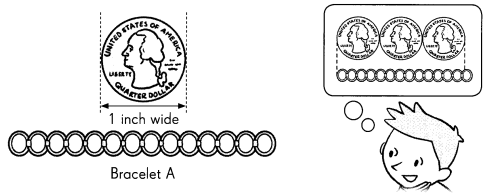Question 9.
Bracelet A is about _________ quarters long.
Bracelet A is about 3 quarters long.

Question 10.
It is about ___________ inches long.
It is about 3 inches long.
Explanation:
In the above image we can observe one quarter is 1 inch wide. There are 3 quarters. It is about 3 inches long.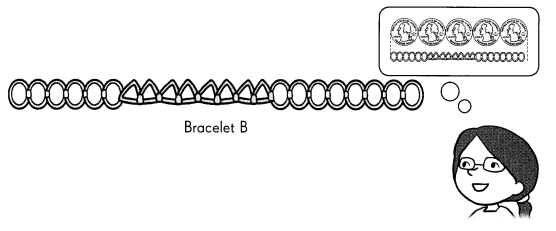Question 11.
Bracelet B is about ___________ quarters long.
Bracelet B is about 5 quarters long.

Question 12.
It is about __________ inches long.
It is about 5 inches long.
Explanation:
In the above image we can observe one quarter is 1 inch wide. There are 5 quarters. It is about 5 inches long.

Estimate the length of each object to the nearest half-inch.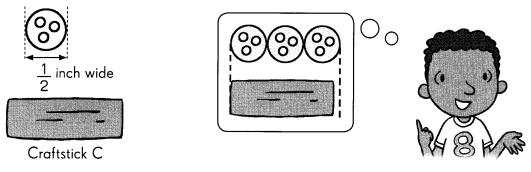Question 13.
Craft stick C is about ___________ buttons long.
Craft stick C is about 3 buttons long.

Question 14.
It is about ___________ inches long.
It is about 1 1/2 inches long.
Explanation:
In the above image we can observe one button is 1/2 inch wide. There are 3 buttons. It is about 1 1/2 inches long.

Estimate the length of each object to the nearest half-inch.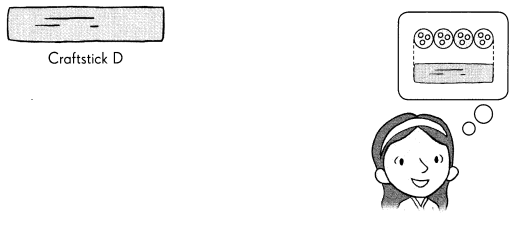Question 15.
Craft stick D is about ___________ buttons long.
Craft stick D is about 4 buttons long.

Question 16.
It is about ___________ inches long.
It is about 2 inches long.
Explanation:
In the above image we can observe one button is 1/2 inch wide. There are 4 buttons. It is about 2 inches long.

Fill in the blanks. These are 12-inch rulers.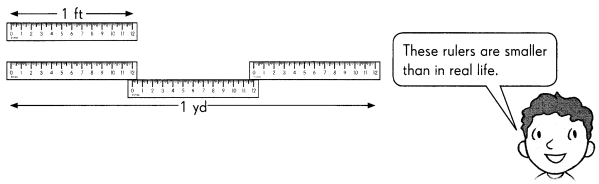Question 17.
1 ft = ____________ in.
1 feet = 12 inch

Question 18.
1 yd = ___________ ft = ___________ in.
1 yard = 3 feet = 36 inches
Explanation:
In the above image we can observe 1 feet is equal to 12 inches. We know that 1 yard is equal to 3 feet.
3 x 12 = 36 inches
3 feet is equal to 36 inches.

Name 3 objects that are 1 foot long each.

Question 19.
The 3 items are ___________, ___________, and ___________.

Name 3 objects that are longer than 1 foot but shorter than 3 feet.

Question 20.
___________, ___________, and ___________ are longer than 1 foot but shorter than 3 feet.

Sarah is going on a treasure hunt. She is looking for objects that are about 3 feet long. 3 feet is equal to 1 yard.

Look at the objects she has found. Then sort them in the table.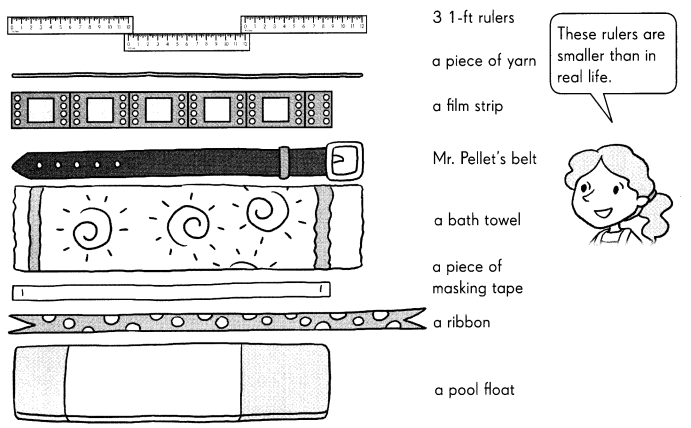Question 21.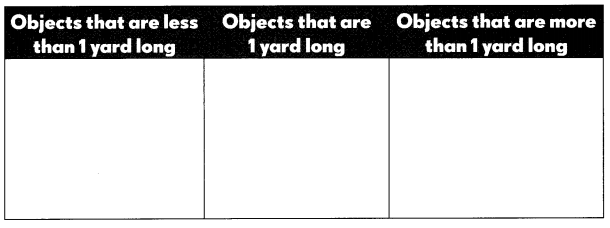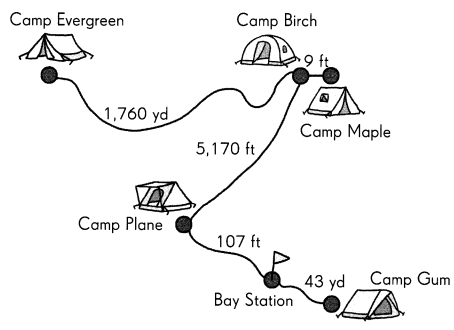Question 22.
The distance between Camp Evergreen and Camp Birch is __________ mile.
The distance between Camp Evergreen and Camp Birch is 1 mile.
Explanation:
In the above image we can observe the distance between Camp Evergreen and Camp Birch is 1,760 yard. We know that 1 mile is equal to 1,760 yard.

Question 23.
The distance between Camp Birch and the Bay Station is about __________ mile.
The distance between Camp Birch and the Bay Station is about 1 mile.
Explanation:
The distance between camp Birch to camp plane is 5,170 feet.
The distance between camp plane to bay station is 107 feet.
5,170 feet + 107 feet = 5,277 feet
1 mile = 5,280 feet

Question 24.
The distance between Camp Birch and Camp Maple is __________ yards.
The distance between Camp Birch and Camp Maple is 3 yards.
Explanation:
The distance from camp Birch to camp Maple is 9 feet.
1 yard = 3 feet
2 yards = 6 feet
3 yards = 9 feet

Question 25.
Camp __________ is nearer to the Bay Station than Camp Gum.
Camp Plane is nearer to the Bay Station than Camp Gum.

Question 26.
The distance between Camp Plane and Camp Birch is slightly less than 1,760 ___________.

Choose the unit that you would use to measure each. Write inch, foot, yard, or mile.

Question 27.
The length of a hiking trail ___________